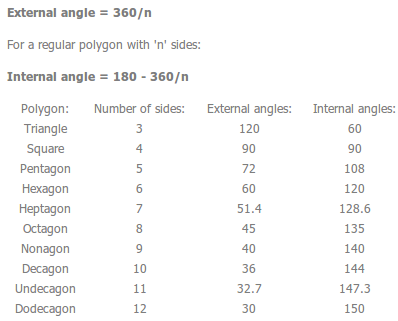# Polygons, Circles, Transformations & Data

?
• Created by: Kathryn
• Created on: 10-05-14 09:17

## Polygons1 of 9

## Special Types of Triangles

Equilateral - All 3 sides equal and all 3 angles equal (to 600).

Isosceles - Two sides the same and the angles at the bottom of those sides are equal.

Scalene - No sides or angles equal.

You can also classify triangles by their angles :

Acute-angled - All angles are acute (less than 900).

Right-angled - One angle is 900.

Obtuse-angled - One angle is obtuse (greater than 900).

The area of a triangle is2 of 9

Square - All sides equal, all angles 90o, 4 lines of symmetry, rotation symmetry of order 4.

Rectangle - Two pais of equal and parallel sides, all angles 90o, two lines of symmetry, rotation symmetry of order 2.

Rhombus - All sides equal, opposite sides parallel, two lines of symmetry, rotation symmetry of order 2. (Basically a square leaning over. Sometimes referred to as a diamond!

Parallelogram - Two pairs of equal and parallel sides, opposite angles equal, no lines of symmetry, rotation symmetry of order 2. (Basically a rectangle leaning over.).

Trapezium - One pair of parallel sides, no symmetry.

Kite - Two pairs of equal sides next to each other, one line of symmetry, no rotation symmetry

3 of 9

## Gathering Data

There are two types of number data: Discrete and Continuous.

Discrete data can only take specific values and not the values in-between.

Continuous data can take any value within your range of accuracy.

Questionnaires

1. Questions must be specific.
2. Questions must not be leading.
4 of 9

## Analysing Data

Mean is the total of all the items divided by the number of items.

Median is the middle value.

Mode is the most common value.

Range is the difference between the smallest and the biggest value.

Grouped data

Mean - assume all the items in a group take the mid-point of the group so for each group you do mid-point x frequency. Add them all up and divide by the Total Frequency.

Median - you cannot find the median, only the group that it is in.

Modal group - the group with the highest frequency.

Note: if there are an even number of items then there will be two middle values so the median will be halfway between them.

5 of 9

## Pie Charts

To work out the angle needed for each section:To find out the frequency that each section represents, measure the angle for the section then:6 of 9

## Other Charts/Diagrams

Frequency Diagrams

These are sometimes called bar charts.

These are a good way of looking at the spread of data and are very easy to draw.

Line Graphs

Line Graphs are only used for discrete data and are simply a line (instead of a bar) for each data value showing total frequency.

## Scatter Diagrams

Simply plot crosses on a graph for the two things you are looking at.

If the data follows a 'trend' or 'correlation' we can draw a line of best fit showing the general slope of the data.

You can then get further information from the graph by using your line of best fit.

7 of 9

## Cumulative Frequency

Key Points

1. Cumulative frequency simply means adding the frequencies up as you go along.
2. When plotting the graph, always plot points using the upper value of each group.
3. Cumulative frequency is always plotted on the vertical axis (up the side) and the range of data goes across the bottom.
4. The shape of a cumulative frequency curve looks like a 'stretched S' and is called an ogive.

The more 'stretched-out' the 'S' is the more spread out the data is. An 'S' with a very steep middle section indicates the data being tightly grouped around the median.

1. Median - go halfway up the cumulative frequency axis, read across and down then read the median from the bottom scale.
2. Lower Quartile - go a quarter of the way up the cumulative frequency axis, read across and down then read the Lower Quartile from the bottom scale.
3. Upper Quartile - go three-quarters of the way up the cumulative frequency axis, read across and down then read the Upper Quartile from the bottom scale.
4. Inter-Quartile Range - the difference between the Lower Quartile and the Upper Quartile.
8 of 9

## Transformations & Similar Shapes

1. Reflection - mirror line.
2. Rotation - angle, direction, centre.
3. Enlargement - scale factor, centre.
4. Translation - vector.

Similar shapes are enlargements of one another. Similar shapes have the same angles and sizes proportionate.This is not the same as another tricky maths word - congruent. Two shapes are congruent if they are exactly the same! Same sides, same angles, same everything!

1. Always use corresponding sides when you work out the scale factor.

2. Don't forget that in enlargements the angles don't change!

9 of 9